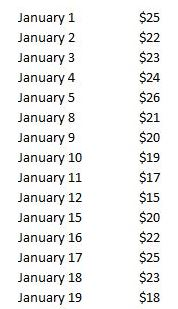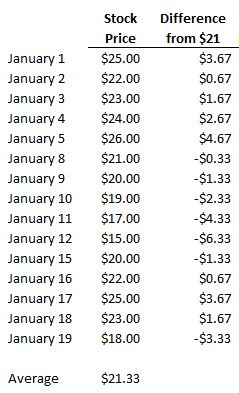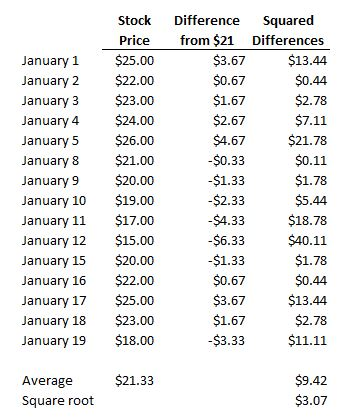# Variability

Updated July 11, 2021

## What is Variability?

Variability is the degree to which a data series deviates from its mean (or in the accounting world, how much a budgeted value differs from an actual value).

## How Does Variability Work?

For example, let's say Company XYZ stock has the following prices:The average of these prices is \$21.33. To calculate the variance, we see how "far away" each day's stock price is from \$21.33, like this:Notice that some of the differences are negative. Because we're going to calculate the average difference, the negative numbers create a mathematical problem (they'll offset the positive numbers and screw up the calculation). To avoid this, we square each difference so that each difference is positive, like this:The last step is simply calculating the average of those squared differences, which is \$9.42, and then taking the square root of that number to get the amount by which Company XYZ stock tends to vary from its average price.

The square root is \$3.07, meaning that when Company XYZ deviates from that \$21 average, it tends to do so by about \$3.07.

## Why Does Variability Matter?

This is only one way to measure variability. Beta, regression analysis, and many other statistical methods are designed to figure out just how volatile a data series is. Variability is a measure of volatility and thus a measure of risk, because it measures how much something like a stock tends to deviate from its "usual" value. The higher the variability, the more wildly the stock fluctuates when it fluctuates. Accordingly, the higher the variability, the riskier the stock.

All of our content is verified for accuracy by Paul Tracy and our team of certified financial experts. We pride ourselves on quality, research, and transparency, and we value your feedback. Below you'll find answers to some of the most common reader questions about Variability.
##### Be the first to ask a question

Paul Tracy - 140Paul has been a respected figure in the financial markets for more than two decades. Prior to starting InvestingAnswers, Paul founded and managed one of the most influential investment research firms in America, with more than 2 million monthly readers.